# KSEEB Solutions for Class 5 Maths Chapter 8 Symmetrical Figures

Students can Download Maths Chapter 8 Symmetrical Figures Questions and Answers, Summary, Notes Pdf, KSEEB Solutions for Class 5 Maths helps you to revise the complete Karnataka State Board Syllabus and score more marks in your examinations.

## Karnataka State Syllabus Class 5 Maths Chapter 8 Symmetrical Figures

### KSEEB Class 5 Maths Symmetrical Figures Ex 8.1

1. Mark symmetric figures as (✓) and non symmetric figure as (✗) in the following: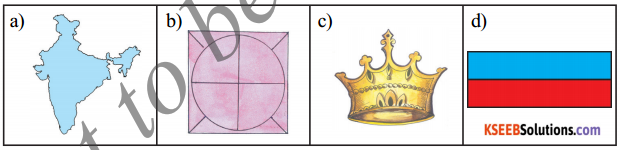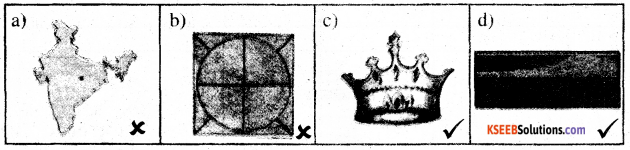2. A few lines have been drawn on the following figures and the lines are denoted by numbers. Identify the axes of symmetry and write its number.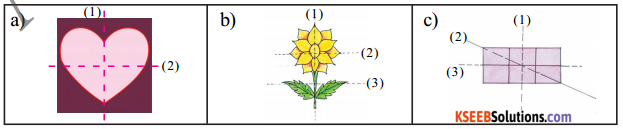a) (2), b) (1), c) (1) ಮತ್ತು (3)3. Draw axes of symmetry to the following symmetrical figures.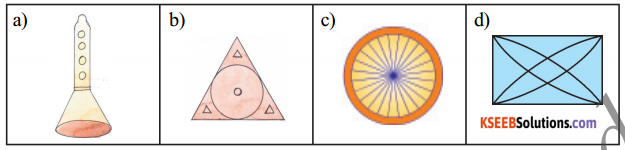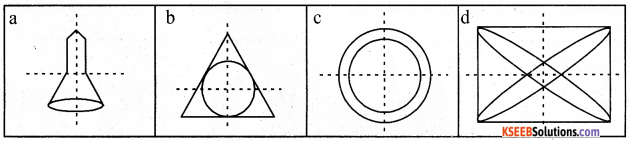4. A few symmetrical figures with only half part are given below. Complete the remaining half part by drawing.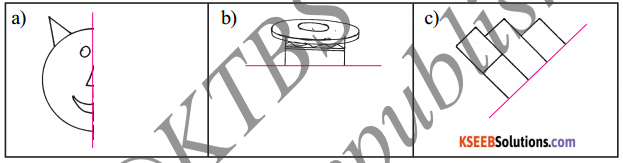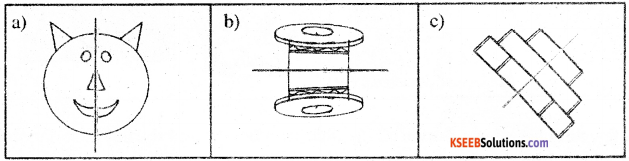5. Pairs of figures are given below. If one figure is the image of the other figure put a tick (✓) if not put (✗).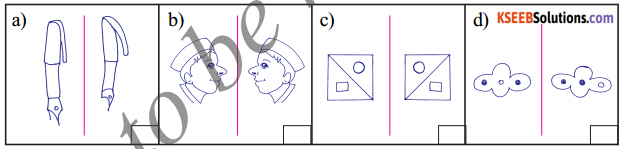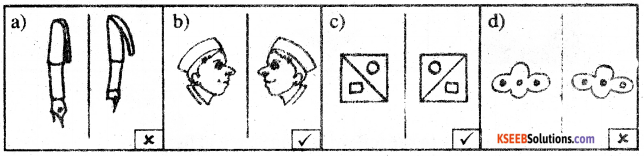6. Some figures are given below. Draw their images in the given space.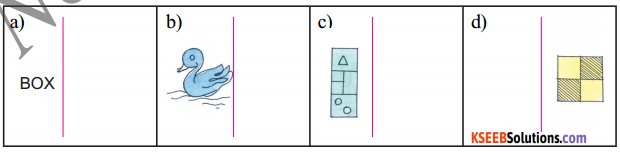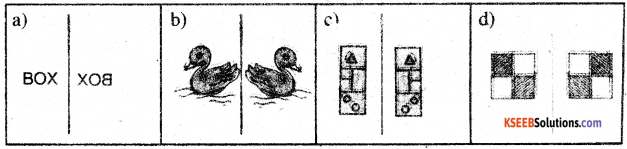7. A few numerals and words are given below. Among them a few numerals or words are the images of original numerals and words. List them.
a) 818
b) 310
c) 18081
d) 80888
f) MAM
g) EVE
h) THAT
a) 818 c) 1808 f) MAM

8. List the symmetrical objects you have seen in your surroundings.
Paper, Mobile, Chair, lock, ball### KSEEB Class 5 Maths Symmetrical Figures Ex 8.2

1. Figures are given in the first row’ of the table given below. In the next rows, the rotations it has to be turned are given. If the figure is turned forgiven rounds and resembles the original figure, put (✓) in the box and if it does not resemble put (✗) in the box.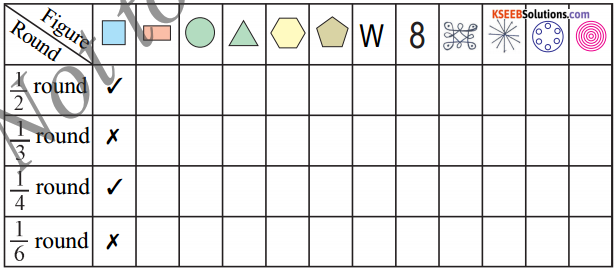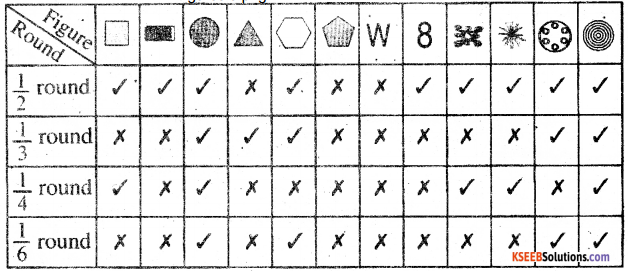2. The follow ing figures when turned $$\frac{1}{3}$$ round do not resemble the original figure. Make suitable changes in the figure, to make them resemble the original figure when turned $$\frac{1}{3}$$ round.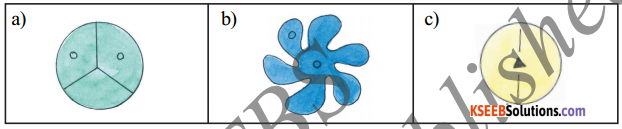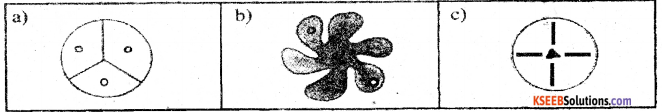3. a) List the letters from English alphabet, which will not change their shape, when turned $$\frac{1}{2}$$ rotation.

Example: X
H, I, N, O, S, Z.

b) Which capital letter from English alphabet when turned $$\frac{1}{4}$$ round gives another letter?
N

c) Which capital letter from English alphabet when rotated $$\frac{1}{2}$$ round gives another letter?
M.

4. Draw the figures which resemble the original figure when rotated $$\frac{1}{4}$$ round.
5. Draw the figures which resemble the original figure when rotated $$\frac{1}{3}$$ round.